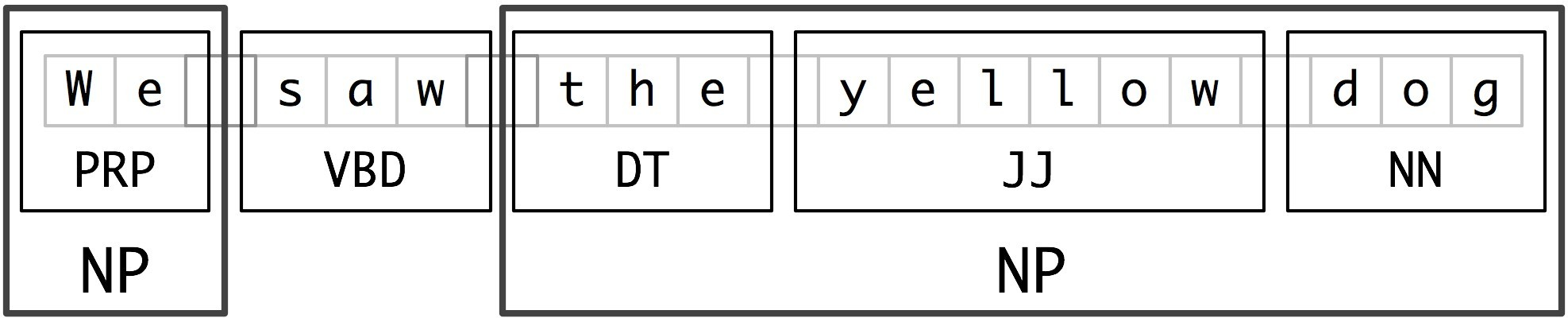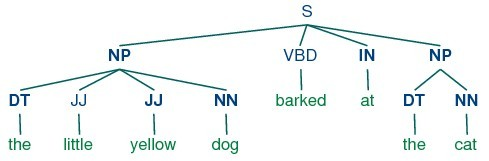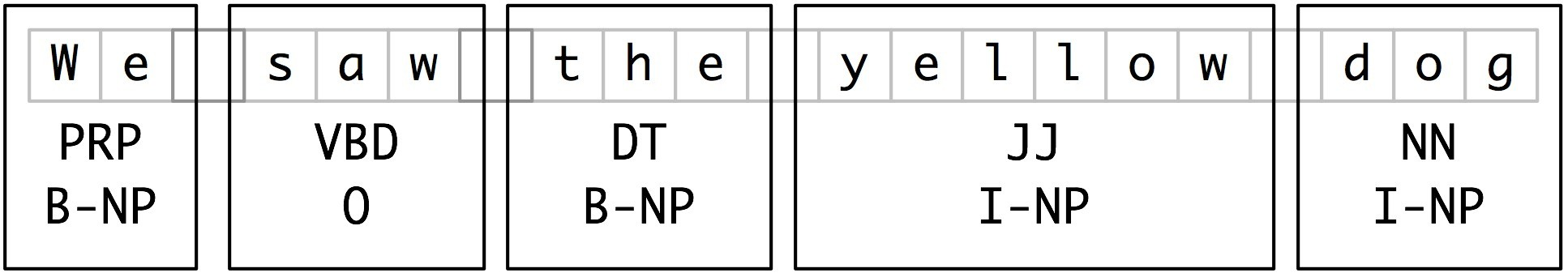# Python自然语言处理学习笔记(54)：朴素贝叶斯分类器

6.5   Naive Bayes Classifiers 朴素贝叶斯分类器

In naive Bayes classifiers, every feature gets a say in determining which label should be assigned to a given input value. To choose a label for an input value, the naive Bayes classifier begins by calculating the prior probability（先验概率） of each label, which is determined by checking frequency of each label in the training set. The contribution from each feature is then combined with this prior probability, to arrive at a likelihood estimate for each label. The label whose likelihood estimate is the highest is then assigned to the input value. Figure 6.14 illustrates this process.Figure 6.14: An abstract illustration of the procedure used by the naive Bayes classifier to choose the topic for a document. In the training corpus, most documents are automotive, so the classifier starts out at a point closer to the "automotive" label. But it then considers the effect of each feature. In this example, the input document contains the word "dark," which is a weak indicator for murder mysteries, but it also contains the word "football," which is a strong indicator for sports documents. After every feature has made its contribution, the classifier checks which label it is closest to, and assigns that label to the input.

Individual features make their contribution to the overall decision by "voting against" labels that don't occur with that feature very often. In particular, the likelihood score for each label is reduced by multiplying it by the probability that an input value with that label would have the feature. For example, if the word run occurs in 12% of the sports documents, 10% of the murder mystery documents, and 2% of the automotive documents, then the likelihood score for the sports label will be multiplied by 0.12; the likelihood score for the murder mystery label will be multiplied by 0.1, and the likelihood score for the automotive label will be multiplied by 0.02. The overall effect will be to reduce the score of the murder mystery label slightly more than the score of the sports label, and to significantly reduce the automotive label with respect to the other two labels. This process is illustrated in Figure 6.15 and Figure 6.16.Figure 6.15: Calculating label likelihoods with naive Bayes. Naive Bayes begins by calculating the prior probability of each label, based on how frequently each label occurs in the training data. Every feature then contributes to the likelihood estimate for each label, by multiplying it by the probability that input values with that label will have that feature. The resulting likelihood score can be thought of as an estimate of the probability that a randomly selected value from the training set would have both the given label and the set of features, assuming that the feature probabilities are all independent.

Underlying Probabilistic Model 潜在的概率模型

Another way of understanding the naive Bayes classifier is that it chooses the most likely label for an input, under the assumption that every input value is generated by first choosing a class label for that input value, and then generating each feature, entirely independent of every other feature. Of course, this assumption is unrealistic; features are often highly dependent on one another. We'll return to some of the consequences（结果） of this assumption at the end of this section. This simplifying assumption, known as the naive Bayes assumption (or independence assumption 独立性假设) makes it much easier to combine the contributions of the different features, since we don't need to worry about how they should interact with one another.Figure 6.16: A Bayesian Network Graph illustrating the generative process（生成过程） that is assumed by the naive Bayes classifier. To generate a labeled input, the model first chooses a label for the input, then it generates each of the input's features based on that label. Every feature is assumed to be entirely independent of every other feature, given the label.

Based on this assumption, we can calculate an expression for P(label|features), the probability that an input will have a particular label given that it has a particular set of features. To choose a label for a new input, we can then simply pick the label l that maximizes P(l|features).

To begin, we note that P(label|features) is equal to the probability that an input has a particular label and the specified set of features, divided by the probability that it has the specified set of features:

 (2) P(label|features) = P(features, label)/P(features)

Next, we note that P(features) will be the same for every choice of label, so if we are simply interested in finding the most likely label, it suffices to calculate P(features, label), which we'll call the label likelihood.

Note

If we want to generate a probability estimate for each label, rather than just choosing the most likely label, then the easiest way to compute P(features) is to simply calculate the sum over labels of P(features, label):

 (3) P(features) = Σl in| labels P(features, label)

The label likelihood can be expanded out as the probability of the label times the probability of the features given the label:

 (4) P(features, label) = P(label) × P(features|label)

Furthermore, since the features are all independent of one another (given the label), we can separate out the probability of each individual feature:

 (5) P(features, label) = P(label) × Prodf in| featuresP(f|label)`

This is exactly the equation we discussed above for calculating the label likelihood: P(label) is the prior probability for a given label, and each P(f|label) is the contribution of a single feature to the label likelihood.

Zero Counts and Smoothing 零计数和平滑

The simplest way to calculate P(f|label), the contribution of a feature f toward the label likelihood for a label label, is to take the percentage of training instances with the given label that also have the given feature:

 (6) P(f|label) = count(f, label) / count(label)

However, this simple approach can become problematic when a feature never occurs with a given label in the training set. In this case, our calculated value for P(f|label) will be zero, which will cause the label likelihood for the given label to be zero. Thus, the input will never be assigned this label, regardless of how well the other features fit the label.

The basic problem here is with our calculation of P(f|label), the probability that an input will have a feature, given a label. In particular, just because we haven't seen a feature/label combination occur in the training set, doesn't mean it's impossible for that combination to occur. For example, we may not have seen any murder mystery documents that contained the word "football," but we wouldn't want to conclude that it's completely impossible for such documents to exist.

Thus, although count(f,label)/count(label) is a good estimate for P(f|label) when count(f, label) is relatively high, this estimate becomes less reliable when count(f) becomes smaller. Therefore, when building naive Bayes models, we usually employ more sophisticated techniques, known as smoothing techniques（平整法）, for calculating P(f|label), the probability of a feature given a label. For example, the Expected Likelihood Estimation（期望似然估计） for the probability of a feature given a label basically adds 0.5 to each count(f,label) value, and the Heldout EstimationHeldout估计） uses a heldout corpus to calculate the relationship between feature frequencies and feature probabilities. The nltk.probability module provides support for a wide variety of smoothing techniques.

Non-Binary Features 非二元特性

We have assumed here that each feature is binary, i.e. that each input either has a feature or does not. Label-valued features (e.g., a color feature which could be red, green, blue, white, or orange) can be converted to binary features by replacing them with binary features such as "color-is-red". Numeric features can be converted to binary features by binning（回收？）, which replaces them with features such as "4<x<6".

Another alternative is to use regression（回归分析） methods to model the probabilities of numeric features. For example, if we assume that the height（顶点） feature has a bell curve（贝尔曲线） distribution, then we could estimate P(height|label) by finding the mean and variance of the heights of the inputs with each label. In this case, P(f=v|label) would not be a fixed value（定值）, but would vary depending on the value of v.

The Naivete of Independence 独立的朴素

The reason that naive Bayes classifiers are called "naive" is that it's unreasonable（不切实际地） to assume that all features are independent of one another (given the label). In particular, almost all real-world problems contain features with varying degrees of dependence on one another. If we had to avoid any features that were dependent on one another, it would be very difficult to construct good feature sets that provide the required information to the machine learning algorithm.

So what happens when we ignore the independence assumption, and use the naive Bayes classifier with features that are not independent? One problem that arises is that the classifier can end up（结束） "double-counting" the effect of highly correlated features, pushing（促进） the classifier closer to a given label than is justified.

To see how this can occur, consider a name gender classifier that contains two identical features, f1 and f2. In other words, f2 is an exact copy of f1, and contains no new information. When the classifier is considering an input, it will include the contribution of both f1 and f2 when deciding which label to choose. Thus, the information content of these two features will be given more weight than it deserves.

Of course, we don't usually build naive Bayes classifiers that contain two identical features. However, we do build classifiers that contain features which are dependent on one another. For example, the features ends-with(a) and ends-with(vowel) are dependent on one another, because if an input value has the first feature, then it must also have the second feature. For features like these, the duplicated information may be given more weight than is justified by the training set.

The Cause of Double-Counting 双倍计数的原因

The reason for the double-counting problem is that during training, feature contributions are computed separately; but when using the classifier to choose labels for new inputs, those feature contributions are combined. One solution, therefore, is to consider the possible interactions between feature contributions during training. We could then use those interactions to adjust the contributions that individual features make.

To make this more precise, we can rewrite the equation used to calculate the likelihood of a label, separating out the contribution made by each feature (or label):

 (7) P(features, label) = w[label] × Prodf |in| features w[f, label]

Here, w[label] is the "starting score" for a given label, and w[f, label] is the contribution made by a given feature towards a label's likelihood. We call these values w[label] and w[f, label] the parameters or weights（加权） for the model. Using the naive Bayes algorithm, we set each of these parameters independently:

 (8) w[label] = P(label)
 (9) w[f, label] = P(f|label)

However, in the next section, we'll look at a classifier that considers the possible interactions between these parameters when choosing their values.

posted @ 2011-09-03 18:21  牛皮糖NewPtone  阅读(...)  评论(...编辑  收藏